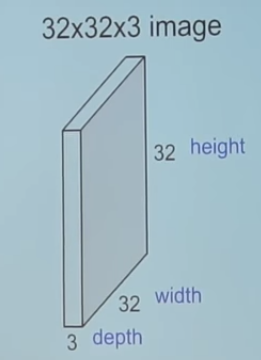The best explanation of Convolutional Neural Networks on the Internet!

CNNs have wide applications in image and video recognition, recommender systems and natural language processing. In this article, the example that I will take is related to Computer Vision. However, the basic concept remains the same and can be applied to any other use-case!

For a quick recap of Neural Networks, here’s a very clearly explained article series.

CNNs, like neural networks, are made up of neurons with learnable weights and biases. Each neuron receives several inputs, takes a weighted sum over them, pass it through an activation function and responds with an output. The whole network has a loss function and all the tips and tricks that we developed for neural networks still apply on CNNs. Pretty straightforward, right?

So, how are Convolutional Neural Networks different than Neural Networks?

CNNs operate over Volumes !

What do we mean by this?1. Example of a RGB image (let’s call it ‘input image’)

Unlike neural networks, where the input is a vector, here the input is a multi-channeled image (3 channeled in this case).

There are other differences that we will talk about in a while.

Before we go any deeper, let us first understand what convolution means.

Convolution

We take the 5*5*3 filter and slide it over the complete image and along the way take the dot product between the filter and chunks of the input image.

For every dot product taken, the result is a scalar.

So, what happens when we convolve the complete image with the filter?

I leave it upon you to figure out how the ‘28’ comes. (Hint: There are 28*28 unique positions where the filter can be put on the image)

Now, back to CNNs

The convolution layer is the main building block of a convolutional neural network.

The convolution layer comprises of a set of independent filters (6 in the example shown). Each filter is independently convolved with the image and we end up with 6 feature maps of shape 28*28*1.

Suppose we have a number of convolution layers in sequence. What happens then?

All these filters are initialized randomly and become our parameters which will be learned by the network subsequently.

I will show you an example of a trained network.

Take a look at the filters in the very first layer (these are our 5*5*3 filters). Through back propagation, they have tuned themselves to become blobs of coloured pieces and edges. As we go deeper to other convolution layers, the filters are doing dot products to the input of the previous convolution layers. So, they are taking the smaller coloured pieces or edges and making larger pieces out of them.

Take a look at image 4 and imagine the 28*28*1 grid as a grid of 28*28 neurons. For a particular feature map (the output received on convolving the image with a particular filter is called a feature map), each neuron is connected only to a small chunk of the input image and all the neurons have the same connection weights. So again coming back to the differences between CNN and a neural network.

CNNs have a couple of concepts called parameter sharing and local connectivity

Parameter sharing is sharing of weights by all neurons in a particular feature map.

Local connectivity is the concept of each neural connected only to a subset of the input image (unlike a neural network where all the neurons are fully connected)

This helps to reduce the number of parameters in the whole system and makes the computation more efficient.

I will not be talking about the concept of zero padding here as the idea is to keep it simple. Interested people can read about it separately!

Pooling Layers

A pooling layer is another building block of a CNN.

Its function is to progressively reduce the spatial size of the representation to reduce the amount of parameters and computation in the network. Pooling layer operates on each feature map independently.

The most common approach used in pooling is max pooling.

Typical Architecture of a CNN

We have already discussed about convolution layers (denoted by CONV) and pooling layers (denoted by POOL).

RELU is just a non linearity which is applied similar to neural networks.

The FC is the fully connected layer of neurons at the end of CNN. Neurons in a fully connected layer have full connections to all activations in the previous layer, as seen in regular Neural Networks and work in a similar way.

I hope you understand the architecture of a CNN now. There are many variations to this architecture but as I mentioned before, the basic concept remains the same. In case you have any doubts/feedback, please comment.